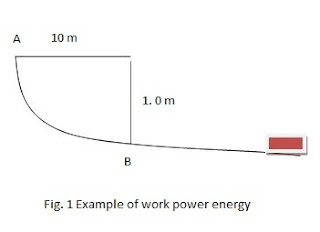Example Of Work Power Energy | Learn Physics

Example 1 of work power energy: A cylindrical tank 10 m high has an internal diameter of 4 m. How much work would be required to fill the tank with water? If water were pumped in  (a)  at the bottom (b) over the top age?

Solution:  Here, D=4 m, r=D/2=4/2=2 m  , h=10 m

Density of water ρ = 103 kg/m3

Mass of water in the tank = πr2 × h × ρ

M= π(2)2 × 10 ×103 =4 π×104 kg.

(a)       When water is pumped in at the bottom, c.g. of water is raised by h = 5 m

Work done, W1 = mgh = 4 π×104×9.8×5 = 6.15 × 106 joule.

(b)   When water is pumped over the top of edge, c.g. has to be raised from h = 0 to h = 10 m (after which it falls itself to height = 5 m).

Work required W2 = mgh’ = 4 π×104×9.8×10 = 12.3 × 106 J

Example 2 of work power energy: In the track shown in fig. 1, section AB is a quadrant of a circle of I m radius. A block is released at A and slides without friction until it reaches point B.

(a)    How fast is it moving at B?

(b)   The horizontal part is not smooth. If the block comes to rest 3.0 n from B, what is the coeff. Of kinetic friction?Solution: Here, r = 1m, v= ?

KE at B = PE at A

(1/2)mv2 = mg(r)

V= 4.43 m/s

Let the block move through a distance s, before coming to rest. Acc. To work energy principle

(1/2)mv2 = F × s = µR × s = µmg × sµ = v2/2gs=(2 ×9.8)/(2  × 9.8 ×3)=0.333